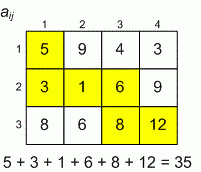Problems

# Turtle

There is a turtle in the left top corner of rectangular table of size n × m. Each cell of the table contains some amount of acid. Turtle can move right or down, its route terminates in right bottom cell of the table.

Each milliliter of acid brings turtle some amount of damage. Find the smallest possible value of damage that will receive a turtle after a walk through the table.

#### Input

First line contains two positive integers n and m, no more than 1000 - the sizes of the table. Then n lines are given, each contains m integers - the description of the table, each number given the amount of acid in the cell (in milliliters).

#### Output

Print the minimum possible damage for the turtle.Time limit 2 seconds
Memory limit 128 MiB
Input example #1
3 4
5 9 4 3
3 1 6 9
8 6 8 12

Output example #1
35

Input example #2
1 1
1

Output example #2
1